# Towards Better Link Prediction in Dynamic Graphs

Companion blog post for Towards Better Evaluation for Dynamic Link Prediction, to appear in NeurIPS 2022 Dataset and Benchmarks Track.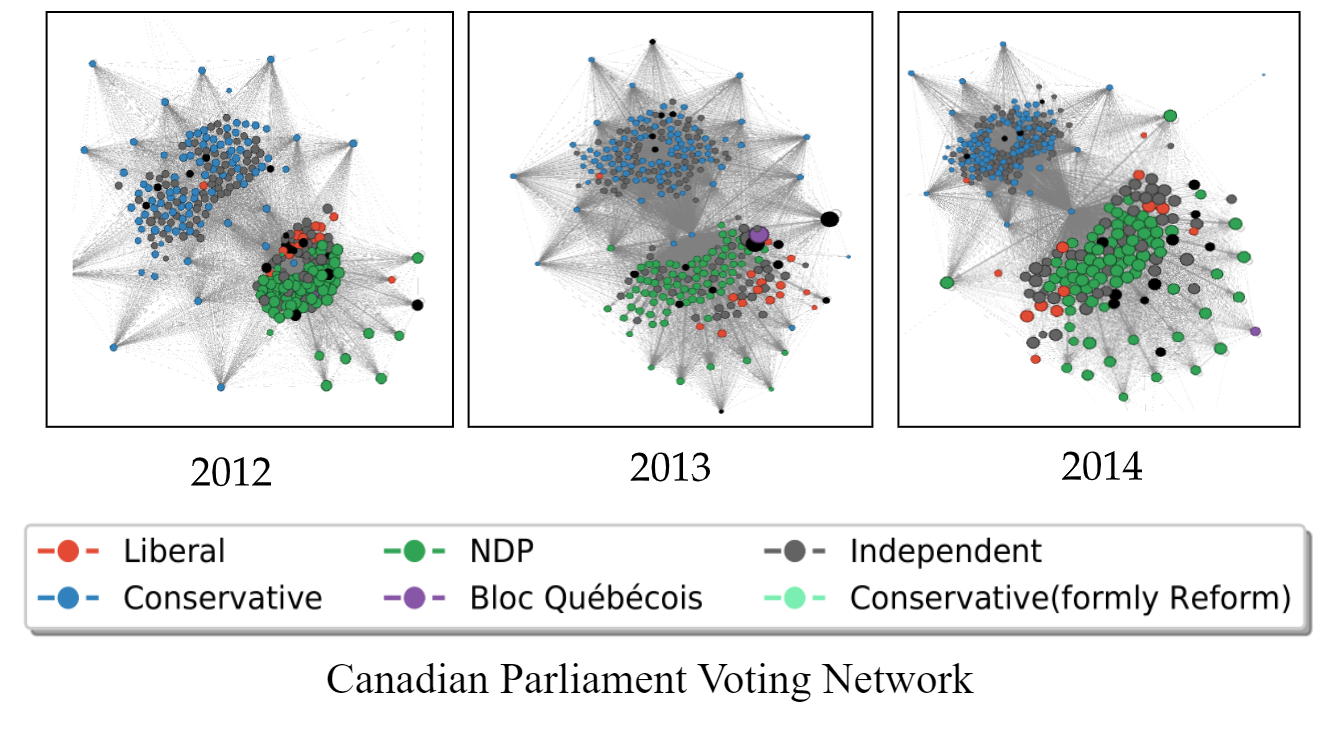A dynamic graph visualization for the Canadian Parliament Voting Network. This is one of the novel benchmark datasets we contributed.

Many real world relations can be formulated as graphs with nodes representing entities and edges being the relations between entities. In a dynamic graph, nodes and edges appear and disappear, while attributes and weights change over time.

Using the above Canadian Parliament voting network as an example, each node represents a Member of Parliament (MP) in the Canadian Parliament and each edge represents that two MPs both voted “yes” on a bill that year. As illustrated, the dynamic graph snapshot varies significantly from 2012 to 2014 and naturally, nodes and edges can be added or deleted over time. In addition, the color of each node reflect which party the MP belongs to and the party affiliation of a given node can also change over time. For those who are interested, the above dataset is publicly available and also incorporated into our proposed benchmark.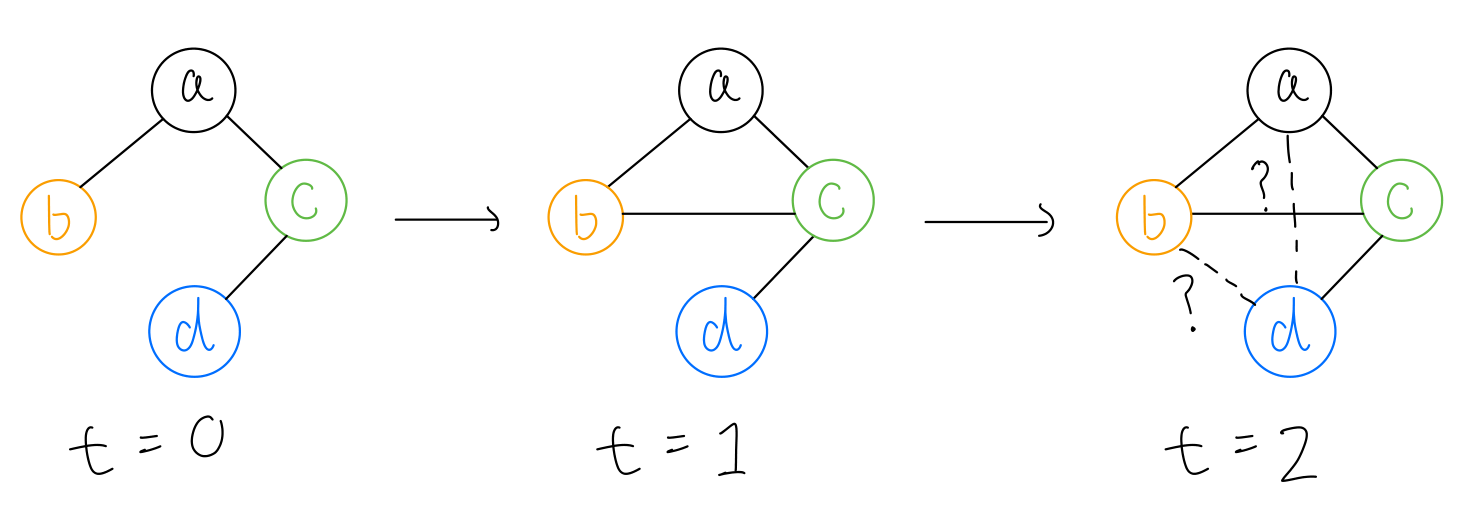An example for dynamic link prediction. Given the graphs at t=0 and t=1, would edges form between (a, d) and (b, d) at time t=2? beside the history of edges, one also need to consider any available node / edge attributes.

A fundamental task on the dynamic graph is the prediction of future interactions between nodes, called dynamic link prediction. More specifically, the goal is to answer this question: at time t in the future, what is the probability p of observing an edge between node i and node j ?Understanding how links are formed are important in social networks, traffic forecasting, political science, recommendation systems and more.

Recently, many methods are proposed for dynamic link prediction and significant progress are made. Some example methods include JODIE , TGN  and CAWN . Based on the existing evaluation setting, close to perfect performance is observed across most methods thus making it harder to differentiate between them. This leads us to closely examine the evaluation settings in dynamic link prediction and rethink how to design more stringent and robust evaluation procedures.

## 💪 Towards Better Evaluation​

First, we identify several limitations in the current evaluation procedure for dynamic link prediction and proposes improvements and additions to address the issues.

• 1️⃣ Limited domain diversity: Existing benchmark datasets are mainly social or interaction networks, thus limited in domain diversity. We introduce 6 new dynamic network datasets from politics, economics, and transportation and proximity domain. We also present novel visualization techniques to better understand the differences between dynamic networks. These visualizations show that a significant portion of the edges reoccurs over time and the reoccurrence pattern vary drastically across domains.

• 2️⃣ Easy negative edges for evaluation: in the standard evaluation setting, negative edges (edges which should be predicted as non-existent) are sampled uniformly at random. Consider the sparsity of edges in real world networks, only a small number of edges are observed among all $N^2$ possible node pairs. Therefore, the edges that have never been observed previously, can be considered as easy negatives. In this work, we design novel negative sampling (NS) strategies to include harder negative edges including historical and inductive NS strategies.

• 3️⃣ Memorization works well: We propose a simple memorization baseline which stores previously observed edges, and then predicts the existence of the edges based on its saved memory. We name this simple scheme EdgeBank, and show that while it requires neither learning nor hyper-parameter tuning, it can achieve strong performance and is a necessary baseline for future methods to compare against.

## ⏳ Understanding Dynamic Graph Datasets​Continuous-time Dynamic graphs can be modelled as a stream of edges

Formally, We consider a (continuous-time) dynamic graph as a timestamped edge stream including triplets of source, destination, and timestamp

$\mathcal{G} = \{(s_1, d_1, t_1), (s_2, d_2, t_2), \ldots \} \mathrm{\: and \:} (0 \leq t_1 \leq t_2 \leq \ldots \leq t_\mathrm{split} \leq T)$

For evaluation purposes, we split the timeline at a point $t_\mathrm{split}$ into all edges appearing before or after that. Therefore, we have train and test set edges ($E_\mathrm{train}$ & $E_\mathrm{test}$). In this regard, edges of a dynamic graph can be categorized as follows:

1. $E_\mathrm{train} \backslash E_\mathrm{test}$: Edges that are only seen during training.

2. $E_\mathrm{train} \cap E_\mathrm{test}$: Edges that are seen during training and reappear during test phase (can be considered transductive edges).

3. $E_\mathrm{test} \backslash E_\mathrm{train}$: Edges that are only seen during test phase(can be considered inductive edges).

First, to better understand the three categories of edges above, we propose novel visualization tools for understanding edge statistics in dynamic graphs.

### 👉 Temporal Edge Appearance (TEA) Plot​

A TEA plot illustrates the portion of repeated edges versus newly observed edges for each timestamp in a dynamic graph.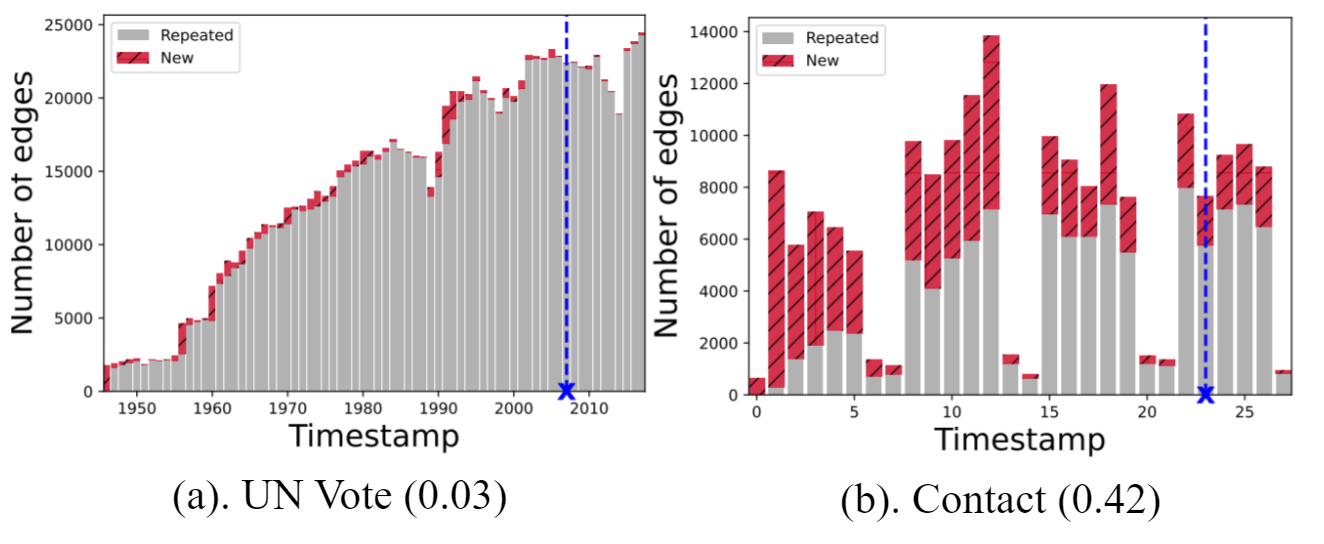The TEA plot shows that real world networks often have a large number of reoccurring edges. The numbers on parentheses reports the **novelty** index.

To quantitatively measure the amount of novel edges seen in a dataset, we propose the novelty index as follows:

$\begin{equation} \mathrm{novelty} = \frac{1}{T} \sum_{t=1}^T \frac{|E_t \backslash E_t^\mathrm{seen}|}{|E_t|} \end{equation}$

where $E_t = {(s, d, t_e) | t_e = t}$ and $E_t^\mathrm{seen} = {(s, d, t_e) | t_e < t}$. We can see that the novelty index varies significantly across datasets and domains (for more TEA plots, see our paper). Therefore, TEA plots imply the importance of the relative distribution of the repeated and new edges when designing temporal graph learning methods.

### 👉 Temporal Edge Traffic (TET) Plot​

A TET plot visualizes the reocurrence pattern of edges in different dynamic networks over time. The edges are sorted based on when they first appeared. To quantify the patterns in the TET plots, we define the following metrics:

\begin{align} \mathrm{reocurrence} &= \frac{|E_\mathrm{train} \cap E_\mathrm{test}|}{|E_\mathrm{train}|} \\ \mathrm{surprise} &= \frac{|E_\mathrm{test} \backslash E_\mathrm{train}|}{|E_\mathrm{test}|} \end{align}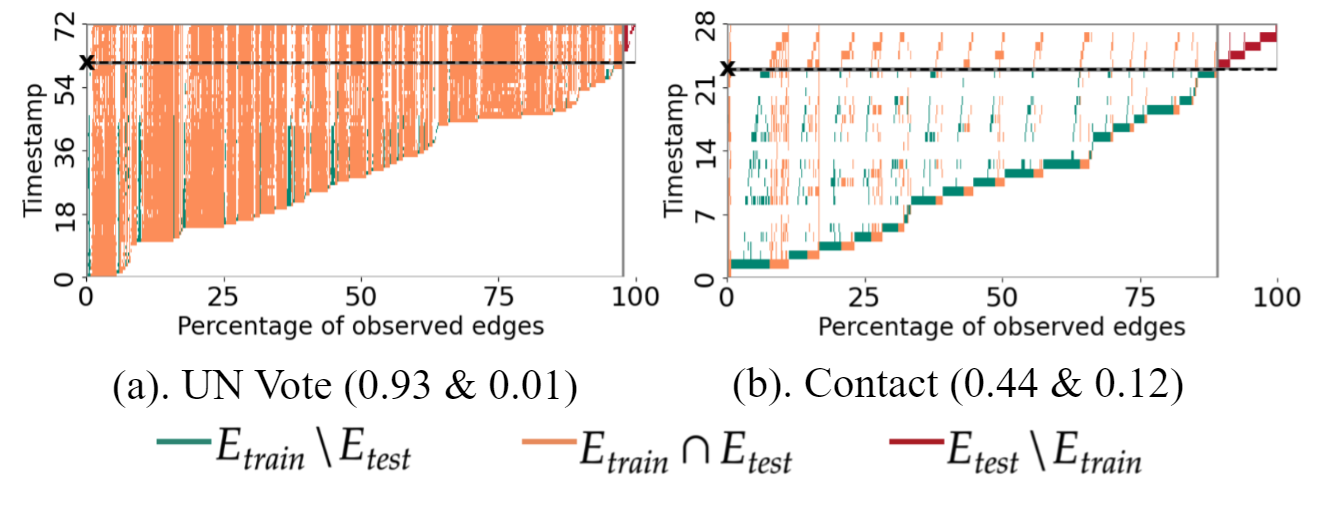TET plots illustrate varied edge traffic patterns in different temporal graphs. The horizontal line starting with “x” marks $t_\mathrm{split}$ In parentheses, we report the proportion of training set edges reoccurring in the test set (reocurrence index) & the proportion of unseen test edges (surprise index)

The reocurrence index reports the proportion of training set edges reoccurring in the test set while the surprise index reports the proportion of unseen test edges. For example, when the surprise index is high, memorization based approaches would work poorly due to the large amount of unseen edges in the test set.

## 🏦 EdgeBank: a Memorization Baseline​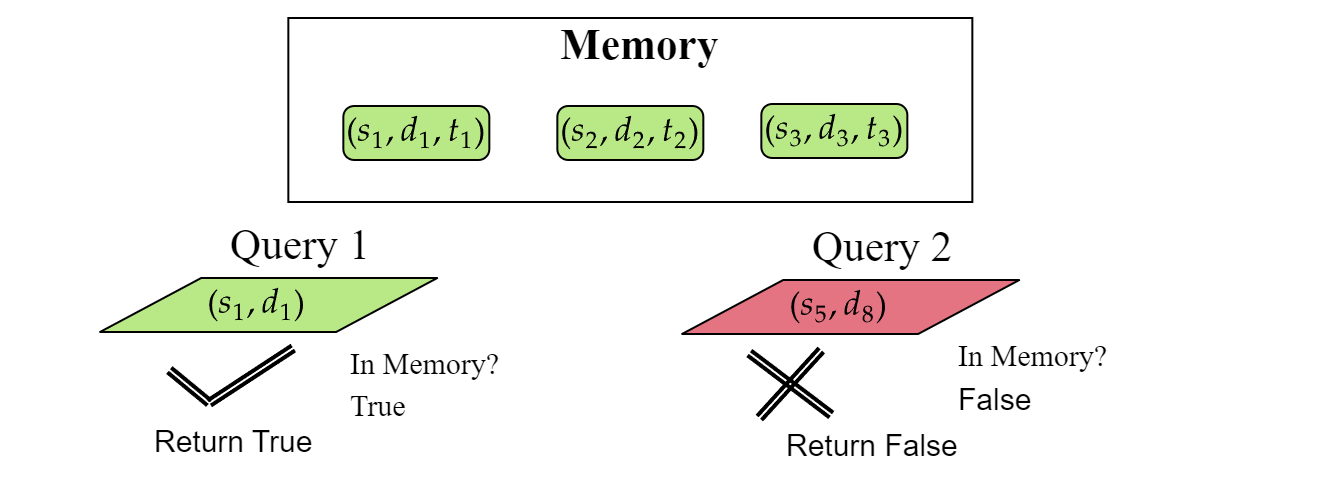Our proposed baseline EdgeBank simply checks if query (s, d) is in memory, if so, return True, else return False

In the previous section, we have seen that many edges in dynamic graphs reoccur over time. Thus, we wondered how far can memorization take you in dynamic link prediction? To answer this question, we propose EdgeBank, a pure memorization baseline which is illustrated above.

EdgeBank consists of a memory component (easily implemented as a hash table), which stores edges observed in the past. At inference time, EdgeBank checks if the query node pair (s, d) is in memory and return True if so, and False otherwise. We observe that while EdgeBank has no learnable parameter or does any learning at all, it achieves surprisingly strong performance in the standard evaluation setting.

## 🔍 Revisiting Negative Sampling​

Motivated by the edge traffic patterns in dynamic graphs, we propose novel, more challenging negative edges for evaluation thus resulting in three categories of negative edges as shown below,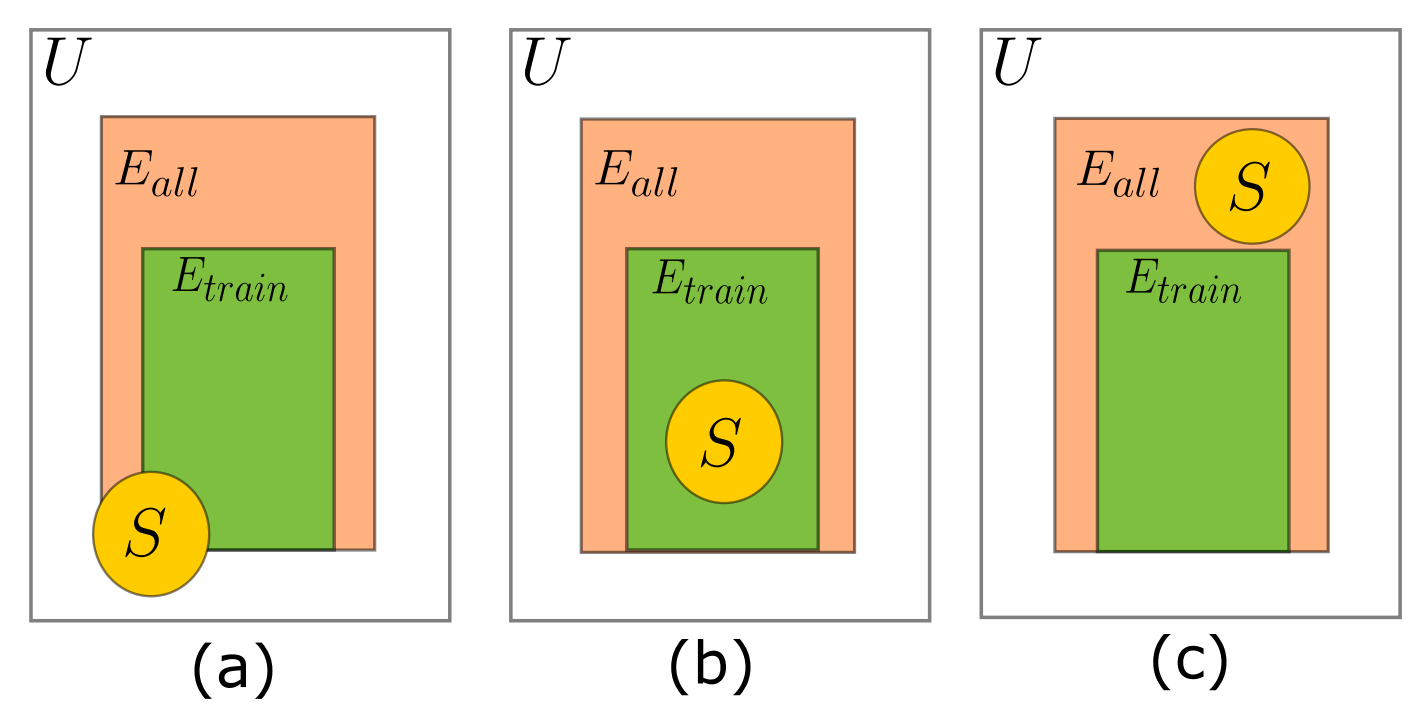Negative edge sampling strategies during evaluation for dynamic link prediction; (a) random sampling (standard in existing works), (b) historical sampling, (c) inductive sampling.
• 1️⃣ Random Negative Sampling: standard sampling procedure in current literature, randomly sample a node pair $(s, d)$.

• 2️⃣ Historical Negative Sampling: sample from the set of edges that have been observed during previous timestamps but are absent in the current step. The objective is to evaluate whether a given method is able to predict in which timestamps an edge would reoccur. Therefore, at time $t$, we sample from the edges $e \in (E_\mathrm{train} \cap E_\mathrm{t})$.

• 3️⃣ Inductive Negative Sampling: the focus here is to evaluate whether a given method can model the reocurrence pattern of edges only seen during test time. Therefore, at time $t$, we sample from the edges $e \in E_\mathrm{test}$ and $e \notin (E_\mathrm{train} \cup E_\mathrm{t})$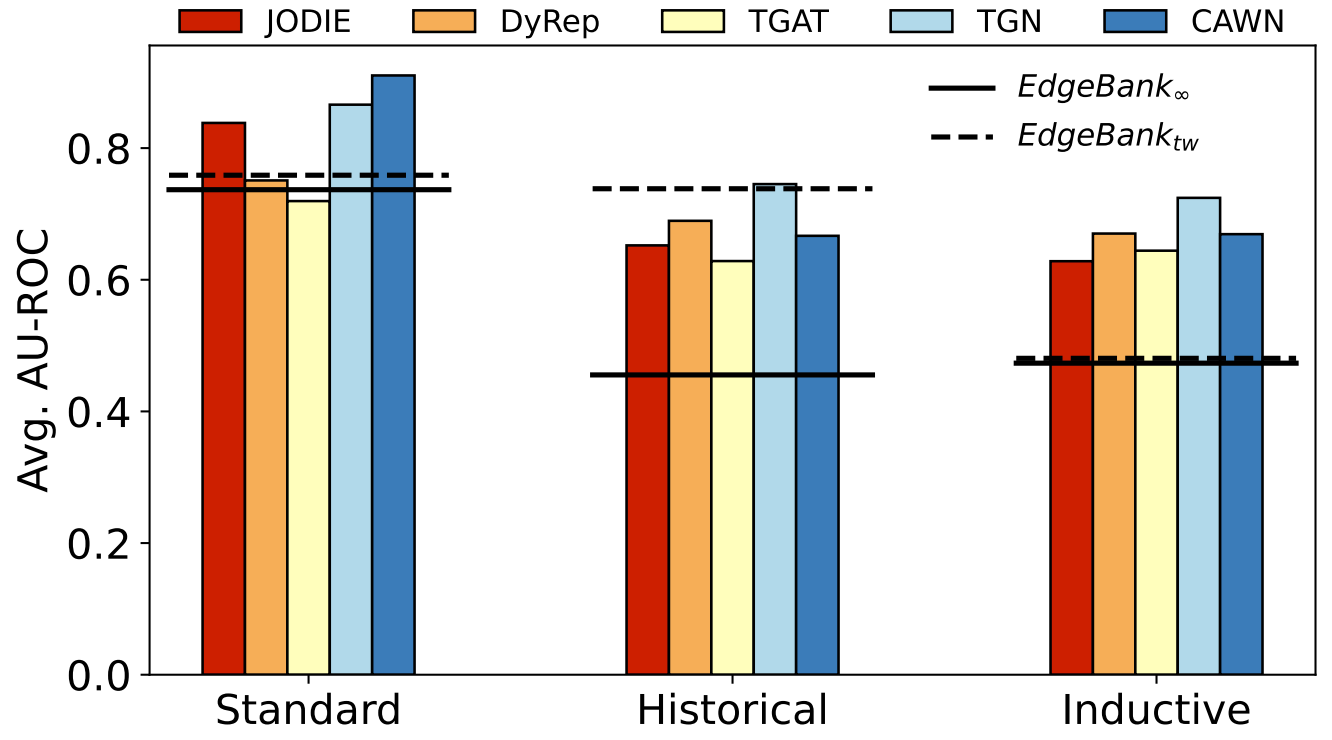The ranking of methods change significantly in different NS settings. Our proposed baselines (horizontal lines) show competitive performance, in particular in the standard setup. The results illustrate the average performance over all datasets studied in our work.

The above Figure shows the performance of each method across the random sampling (standard, left), our proposed historical sampling (middle) and inductive sampling (right) evaluation. Our simple baseline Edgebank achieves strong performance in the standard setting. Also observe that in both historical and inductive setting, all methods have a drop in performance which emphasizes the important of negative sampling during evaluation. In addition, the ordering of methods also change.